# Linear model analysis¶

:

# import relevant module
import openturns as ot
import otpod
# enable display figure in notebook
try:
%matplotlib inline
except:
pass

/calcul/home/dumas/anaconda/lib/python3.6/site-packages/sklearn/ensemble/weight_boosting.py:29: DeprecationWarning: numpy.core.umath_tests is an internal NumPy module and should not be imported. It will be removed in a future NumPy release.
from numpy.core.umath_tests import inner1d


## Generate data¶

:

N = 100
ot.RandomGenerator.SetSeed(123456)
defectDist = ot.Uniform(0.1, 0.6)
# normal epsilon distribution
epsilon = ot.Normal(0, 1.9)
defects = defectDist.getSample(N)
signalsInvBoxCox = defects * 43. + epsilon.getSample(N) + 2.5
# Inverse Box Cox transformation
invBoxCox = ot.InverseBoxCoxTransform(0.3)
signals = invBoxCox(signalsInvBoxCox)


## Run analysis without Box Cox¶

:

analysis = otpod.UnivariateLinearModelAnalysis(defects, signals)

WARNING:root:Some hypothesis tests failed : you may consider to use the Box Cox transformation.


## Get some particular results¶

:

print(analysis.getIntercept())
print(analysis.getR2())
print(analysis.getKolmogorovPValue())

[Intercept for uncensored case : -604.758]
[R2 for uncensored case : 0.780469]
[Kolmogorov p-value for uncensored case : 0.803087]


## Show graphs¶

### The linear model is not correct¶

:

fig, ax = analysis.drawLinearModel()
fig.show()

/calcul/home/dumas/anaconda/lib/python3.6/site-packages/matplotlib/figure.py:459: UserWarning: matplotlib is currently using a non-GUI backend, so cannot show the figure
"matplotlib is currently using a non-GUI backend, "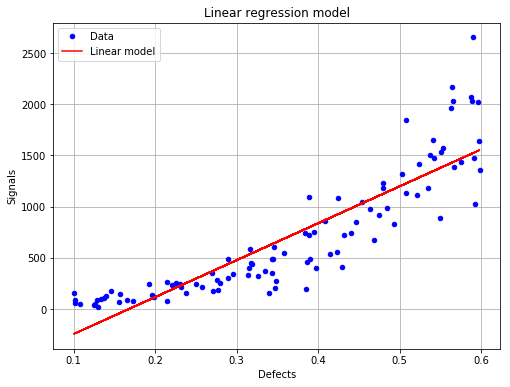### The residuals are not homoskedastic¶

:

fig, ax = analysis.drawResiduals()
fig.show()

/calcul/home/dumas/anaconda/lib/python3.6/site-packages/matplotlib/figure.py:459: UserWarning: matplotlib is currently using a non-GUI backend, so cannot show the figure
"matplotlib is currently using a non-GUI backend, "## Run analysis with Box Cox¶

:

analysis = otpod.UnivariateLinearModelAnalysis(defects, signals, boxCox=True)


## Save all results in a csv file¶

:

analysis.saveResults('results.csv')


## Show graphs¶

### The linear regression model with data¶

:

fig, ax = analysis.drawLinearModel(name='figure/linearModel.png')
# The figure is saved as png file
fig.show()

/calcul/home/dumas/anaconda/lib/python3.6/site-packages/matplotlib/figure.py:459: UserWarning: matplotlib is currently using a non-GUI backend, so cannot show the figure
"matplotlib is currently using a non-GUI backend, "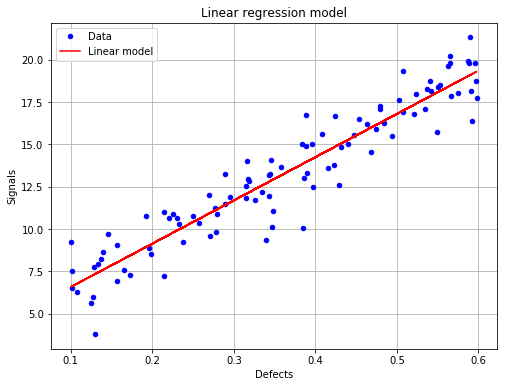### The residuals with respect to the defects¶

:

fig, ax = analysis.drawResiduals(name='figure/residuals.eps')
# The figure is saved as eps file
fig.show()

/calcul/home/dumas/anaconda/lib/python3.6/site-packages/matplotlib/figure.py:459: UserWarning: matplotlib is currently using a non-GUI backend, so cannot show the figure
"matplotlib is currently using a non-GUI backend, "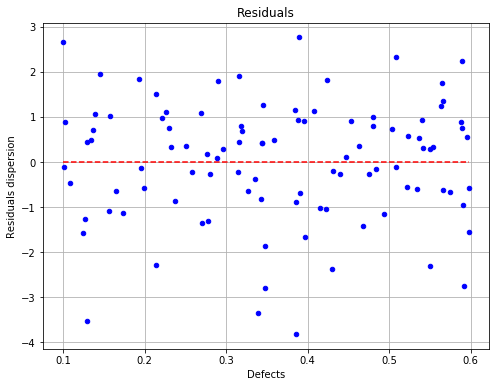### The fitted residuals distribution with the histogram¶

:

fig, ax = analysis.drawResidualsDistribution()
ax.set_ylim(ymax=0.45)
fig.show()
# The figure is saved after the changes
fig.savefig('figure/residualsDistribution.png', bbox_inches='tight')

/calcul/home/dumas/anaconda/lib/python3.6/site-packages/matplotlib/figure.py:459: UserWarning: matplotlib is currently using a non-GUI backend, so cannot show the figure
"matplotlib is currently using a non-GUI backend, "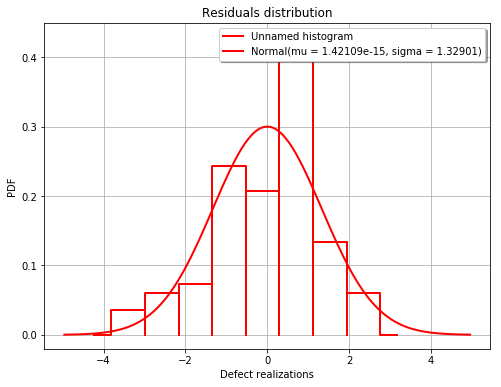### The residuals QQ plot¶

:

fig, ax = analysis.drawResidualsQQplot()
fig.show()

/calcul/home/dumas/anaconda/lib/python3.6/site-packages/matplotlib/figure.py:459: UserWarning: matplotlib is currently using a non-GUI backend, so cannot show the figure
"matplotlib is currently using a non-GUI backend, "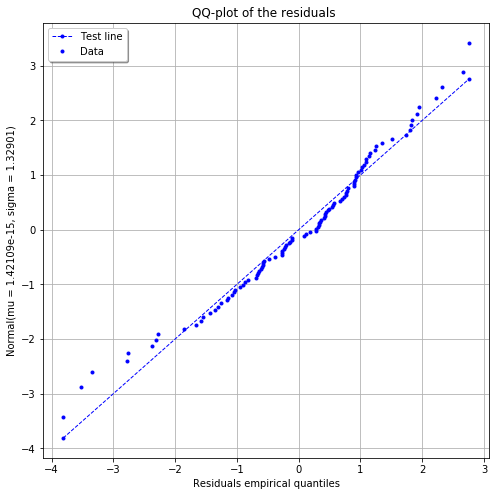### The Box Cox likelihood with respect to the defect¶

:

fig, ax = analysis.drawBoxCoxLikelihood(name='figure/BoxCoxlikelihood.png')
fig.show()

/calcul/home/dumas/anaconda/lib/python3.6/site-packages/matplotlib/figure.py:459: UserWarning: matplotlib is currently using a non-GUI backend, so cannot show the figure
"matplotlib is currently using a non-GUI backend, "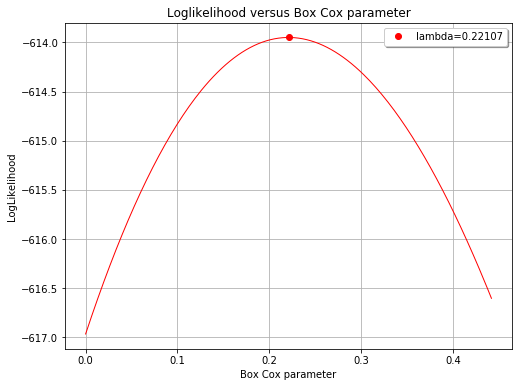[ ]: